# 8.5.1 Alloy (Structured Questions)

Question 1:
Figure 1.1 shows the formation of bronze.(a) On figure 1.1, name the atoms of metals, P and Q. [2 marks]

(b) Name process W. [1 mark]

(c)(i) State one property of bronze. [1 mark]

(ii) State the effect of the atoms of metal Q in bronze. [1 mark]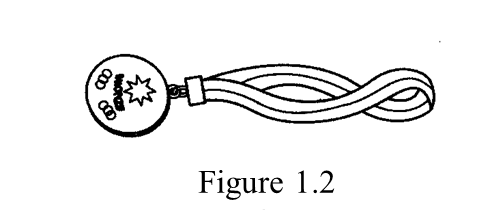(d) The medal in Figure 1.2 is made of bronze.
Give one property of the medal if it is made of metal P only. [1 mark]

(a)
P: Copper
Q: Tin

(b)

Alloying

(c)(i)
Harder/ can withstand corrosion better than copper.

(c)(ii)
The atoms of metal Q in bronze prevent the layers of atoms of metal W in the bronze from sliding easily over one another.

(d)
Softer, more malleable, less resistant to corrosion.

Question 2:
Diagram 2.1 shows an experiment to study the resistance of steel alloy towards corrosion.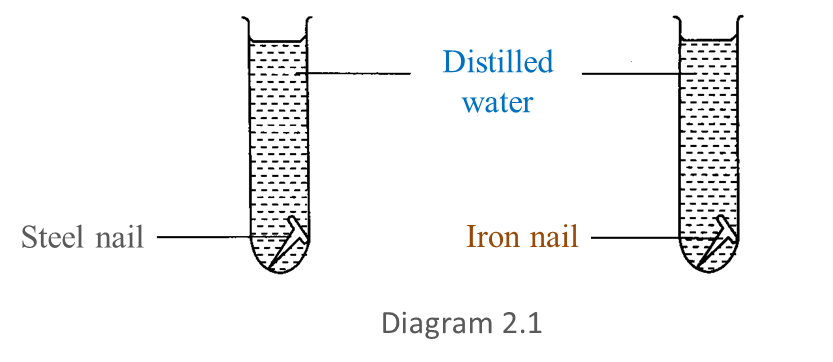After 3 days, the result obtained is shown in Diagram 2.2.(a) Based on Diagram 2.2, write down one observation for this experiment. [1 mark]

(b) Write down one hypothesis for this experiment. [1 mark]

(c) State the variables in this experiment.
(i) Constant variable [1 mark]
(ii) Manipulated variable [1 mark]

(d) Predict the condition of the steel nail on the 5th day. [1 mark]

(e) Based on this experiment, state the operational definition for an alloy. [1 mark]

(a)
The iron nail has corroded while the steel nail has not.

(b)
Steel is more resistant to corrosion than iron.

(c)(i)
Distilled water, number of days the nails are immersed in water.
(any one)

(c)(ii)
Type of metal (steel on iron)

(d)
The steel nail will remain not corroded

(e)
An alloy is a mixture of metal which is harder and more resistant to corrosion than a pure metal.

# 8.4.1 Radio Communication (Structured Questions)

Question 1:
Diagram 1.1 shows three types of electronic components used in a radio receiver.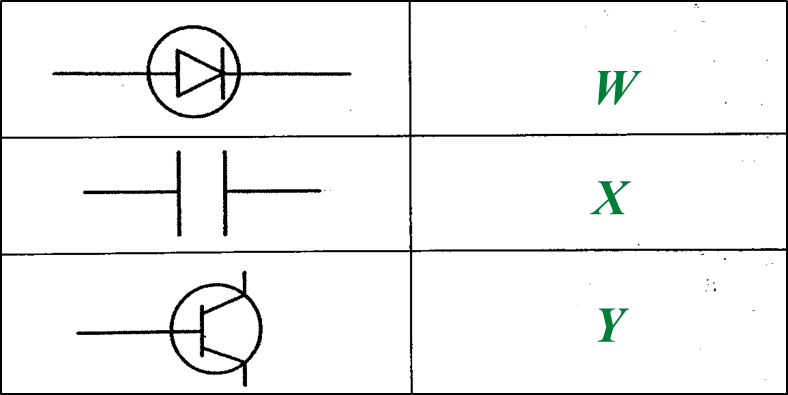Diagram 1.1

(a)
Name electronic components W and Y. [2 marks]

(b)
State the function of electronic component X. [1 mark]

(c)Diagram 1.2

(i)
Write any two electronic components, W, X or Y from Diagram 1.1 into the corresponding blocks provided in Diagram 1.2. [2 marks]

(ii)
What type of wave is received by T?
Mark (\/) your answer in the box provided in Diagram 1.3. [1 mark]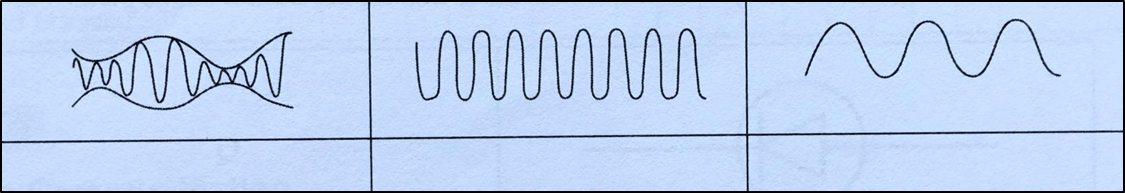Diagram 1.3

(a)
W: Diode
Y: Transistor

(b)
1. Stores electric charges and discharges them at regular intervals when required
2. To channel the flow of radio frequency carrier waves into the Earth.
3. When connected in parallel with the inductor, it determines the frequency of the radio wave that will be detected.

(any one)

(c)(i)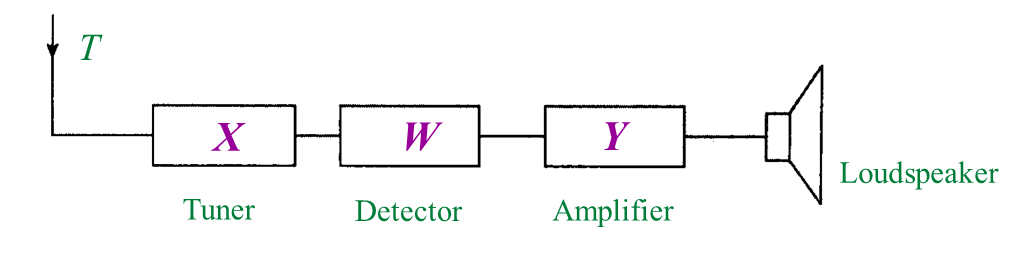(c)(ii)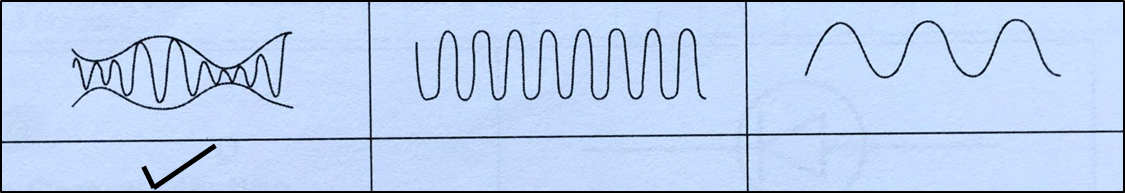Question 2:
Table 1 shows the symbols of electronic components.
(a) Complete Table 1 using the name of electronic components given. [3 marks]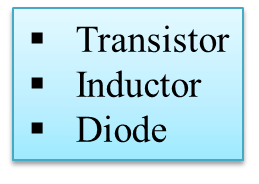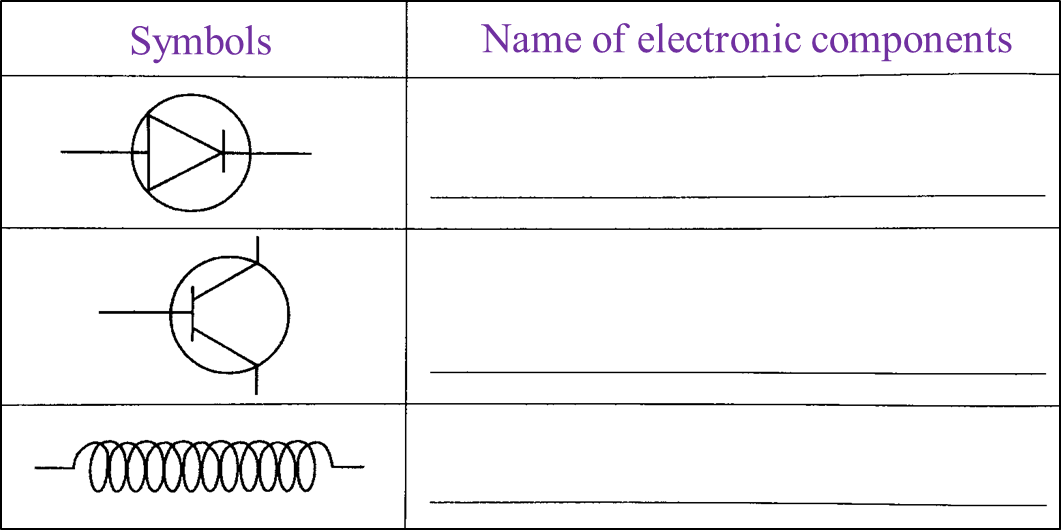Table 1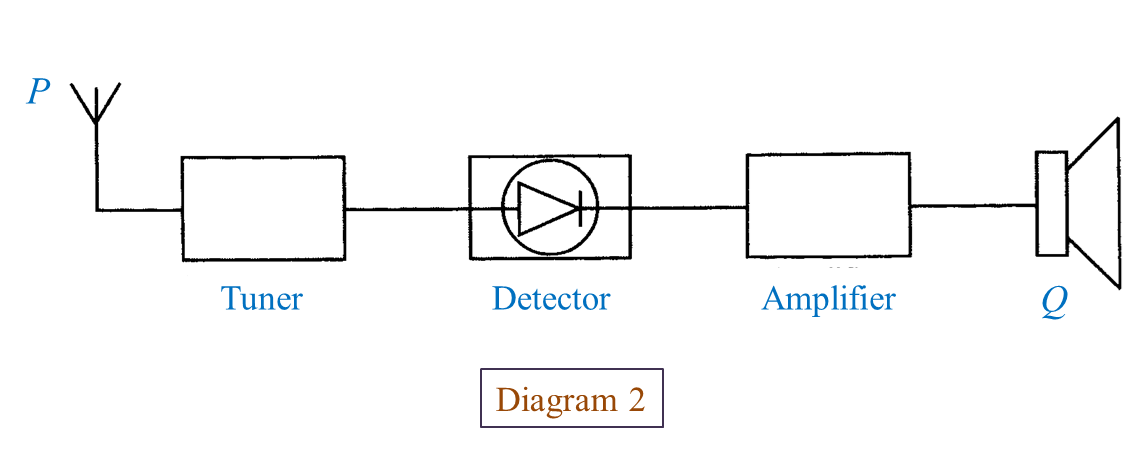(b) What is the function of P? [1 mark]

(c)
What is Q? [1 mark]

(d)
State the energy change that occurs at Q? [1 mark]

(a)(c)(i) Loudspeaker

(c)(ii) Electrical energy → sound energy

# 7.4.3 Practising Responsible Attitudes in the Disposal of Synthetic Polymers (Structured Questions)

Question 1:
Diagram 1 shows containers which are made of synthetic polymer.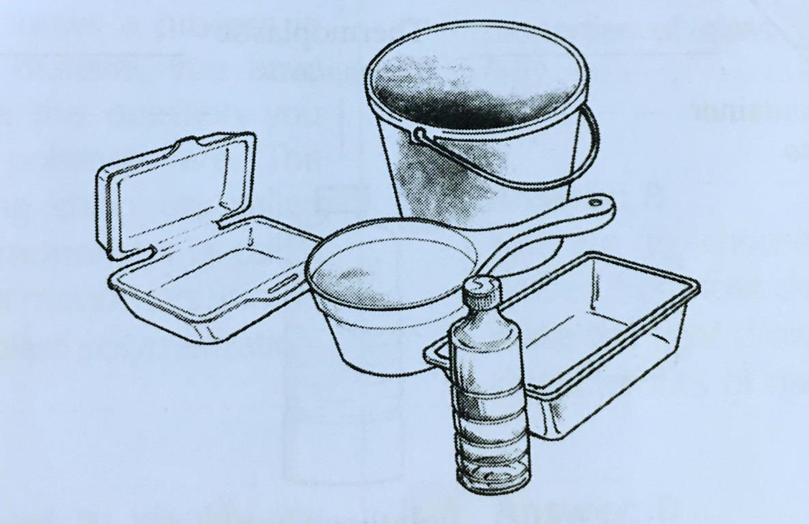Diagram 1

(a) Name the type of the synthetic polymer shown in Diagram 1. [1 mark]

(b)
State two characteristics of the synthetic polymer in Diagram 1. [2 marks]

(c)
What is the effect of improper disposal of the synthetic polymer in Diagram 1 on the environment? [1 mark]

(d)
State two correct ways to dispose of the synthetic polymer in Diagram 1 to preserve the environment. [2 marks]

(a)
Polythene or polyethene

(b)
- Soft when heated and hard when cooled
- Can be moulded more than once

(c)
Improper disposal of the synthetic polymers such as the burning of synthetic polymers releases toxic gases and acidic gases that cause air pollution and acid rain.

(d)
- Recycle or process again
- Burning in special incinerators which does not release toxic gases into the environment

# 7.4.2 Plastics (Structured Questions)

Question 1:
Table 1 shows the characteristics of plastic A and plastic B.Table 1

(a) State the type of plastic A and B. [2 marks]

(b)
State one example of plastic A and B. [2 marks]

(c)
Which plastic can be recycled? [1 mark]

(d)
Which plastic is suitable to make as the handle of an iron? [1 mark]

(a)
A: Thermoplastic
B: Thermosetting plastic (Thermoset)

(b)
A: Perspex
B: Bakelite

(c)
Perspex

(d)
Bakelite

# 7.4.1 Synthetic Polymers (Structured Questions)

Question 1:
Table 1.1 shows two types of product made of rubber.
(a) Complete Table 1.1. [4 marks]Table 1.1

(b) Table 1.2 shows the characteristics of plastic A and plastic B.Table 1.2

In Table 1.2, state the type of plastic A and plastic B. [2 marks]

(a)(b)# 6.3.2 Practising Critical and Analytical Thinking when Selecting Processed Food (Structured Questions)

Question 1:
Diagram 1 shows a labelled container of a processed food.(a) Based on Diagram 1, identify two chemicals written on the label. [2 marks]

(b) Based on the label in Diagram 1, which chemical is used as a preservative? [1 mark]

(c) Name the method of food processing used in Diagram 1. [1 mark]

(d) What other information should be written on the label in Diagram 1 according to Food Regulations 1985?

(a)
1. Benzoic acid
2. Flavouring

(b)

Benzoic acid

(c)

Canning

(d)

- Name of manufacturer

# 6.3.1 The Methods and Substances Used in Food Technology (Structured Questions)

Question 1:
Diagram 1 shows foods that have been processed through three different methods.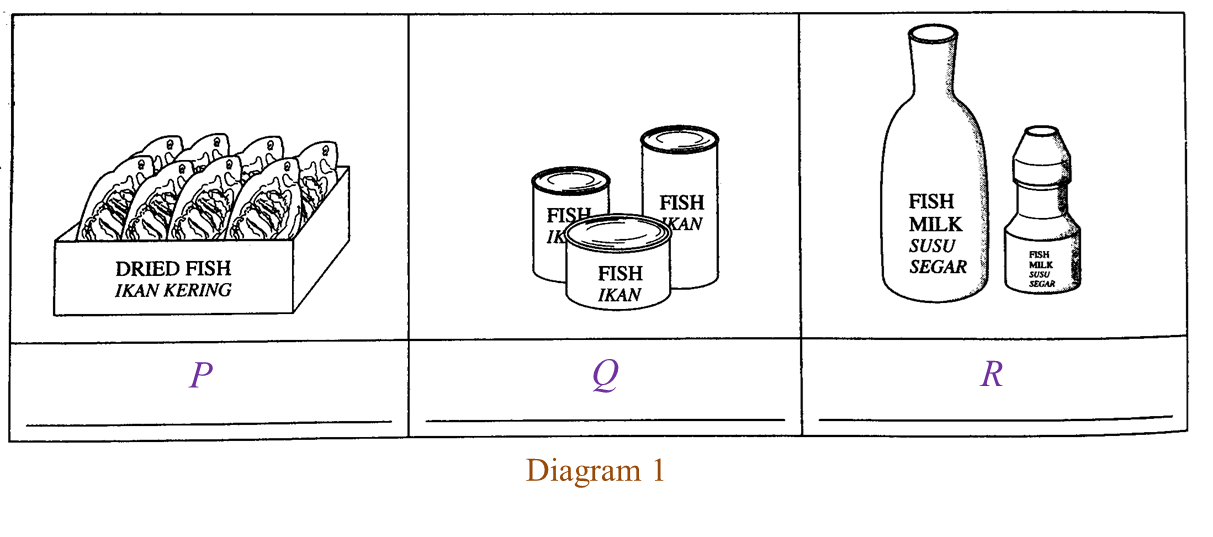(a) Write down the food processing methods, P, Q and R, in Diagram 1. [3 marks]

(b) What happens to the water content in the food that has been processed through method P? [1 mark]

(c) The food is heated at 121 oC during the process in method Q.
What is the purpose of the heating? [1 mark]

(d) Fresh milk is heated for 30 minutes in method R.
What is the temperature used? [1 mark]

(a)
P: Dehydration
Q: Canning
R: Pasteurisation

(b)
Water is removed from the food.

(c)
To kill the microorganisms in the food.

(d)
63 oC

Question 2:
Diagram 2.1 shows a food label of fruit juice that has been processed through a method of food processing according to the Food Regulations 1985.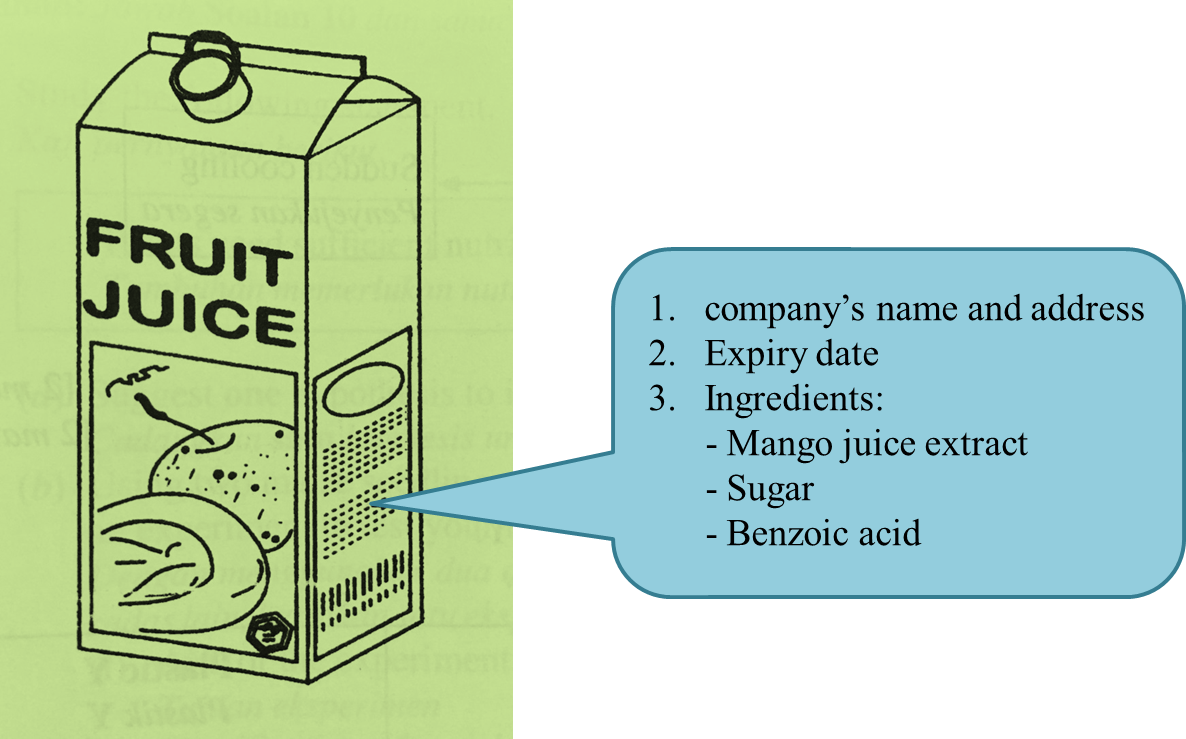Diagram 2.1

(a) Name the preservative added into the fruit juice in Diagram 2.1. [1 mark]

(b) What information is not shown on the food label in Diagram 2.1 as required by the Food Regulations 1985? [1 mark]

(c) Name the food processing method in Diagram 2.1. [1 mark]

(d) Besides fruit juice, state another drink that can be processed by the method in 2(c). [1 mark]

(e) Based on Diagram 2.2, state the temperature and heating duration during the food processing method in 2(c). [1 mark]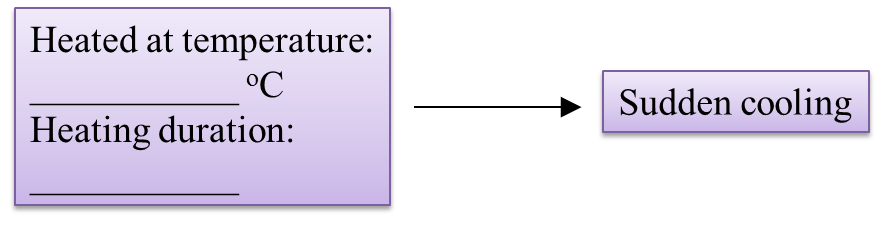Diagram 2.2

(a) Benzoic acid.

(b)
Net weight / Volume of liquid.

(c)
Pasteurization

(d) Milk

(e)
Temperature: 63 oC
Heating duration: 30 minutes
OR
Temperature: 72 oC
Heating duration: 15 seconds

# 5.7.4 The Motion of Vehicles in the Air (Structured Questions)

Question 1:
Diagram 1 shows an experiment to study Bernoulli’s Principle.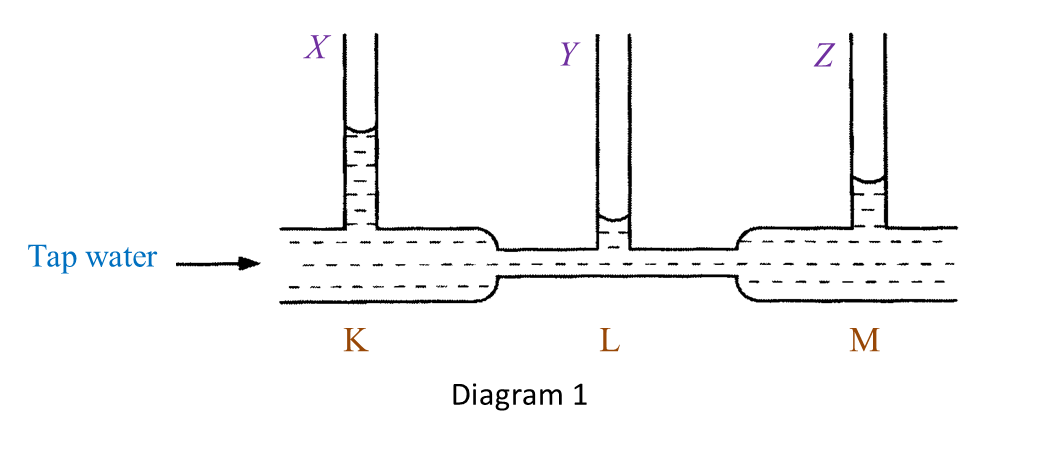(a)(i) State one observation of the water level in Diagram 1. [1 mark]

(ii) State one inference that can be made based on the observation in (a)(i). [1 mark]

(b) State the responding variable in this experiment. [1 mark]

(c) Based on this experiment, state the operational definition for Bernoulli’s Principle. [1 mark]

(d) Mark (\/) in the boxes provided to show the apparatus where the Bernoulli’s Principle is applied. [1 mark]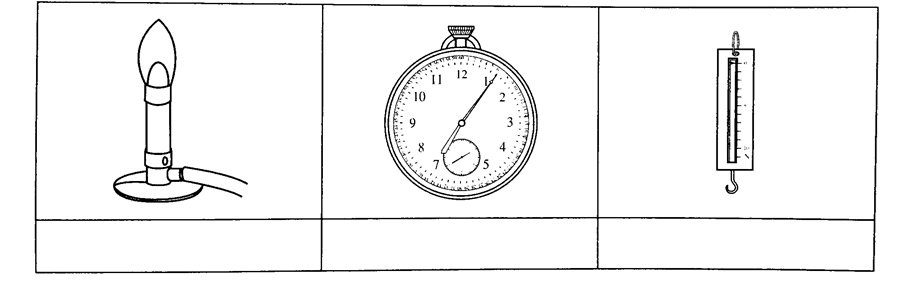(a)(i)
- The water level in the vertical tube Y is the lowest.
- The water level in the vertical tube X is the highest.
(any one)

(a)(ii)
- The water at the base of the vertical tube Y exerts the lowest pressure.
- The water at the base of the vertical tube X exerts the highest pressure.
(any one)

(b)
The pressure exerted by the moving water.

(c)
Bernoulli’s Principle states that pressure in a liquid decreases when the speed of the liquid increases.

(d)# 5.7.3 The Motion of Vehicles in Water (Structured Questions)

Question 1:
Diagram 1 shows an experiment to study the relationship between upthrust and the weight of water displaced.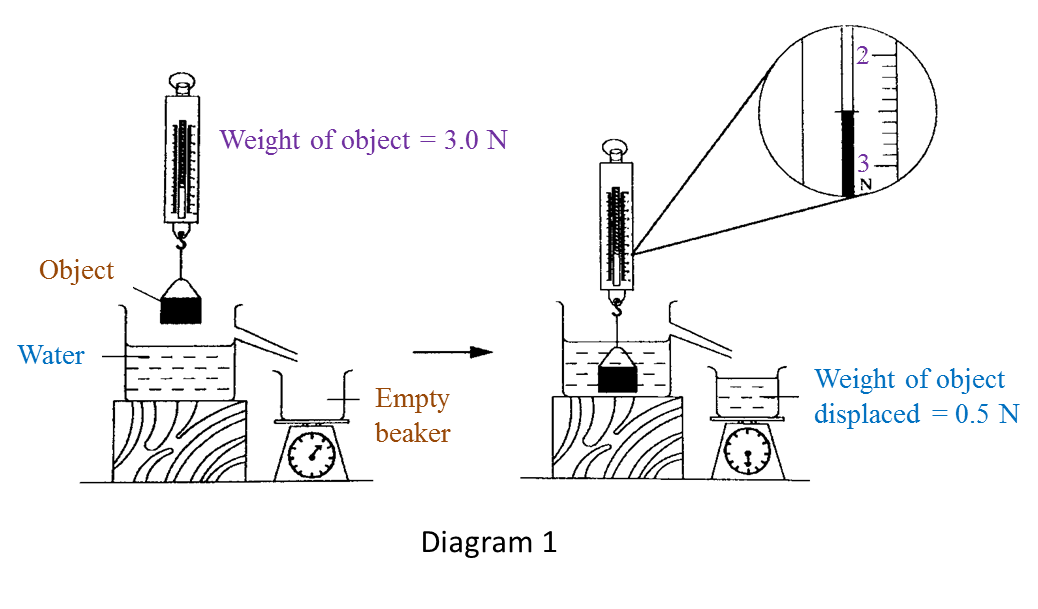(a) Based on Diagram 1, state the weight of object in water and write down your answer in the Table below. [1 mark]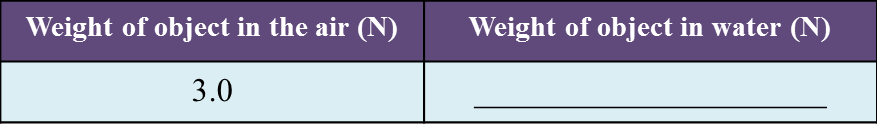(b) Observe Diagram 1. Compare the weight of object in the air and in water. [1 mark]

(c) State one inference for this experiment. [1 mark]

(d) State one responding variable in this experiment. [1 mark]

(e) The upthrust acts on an object when it is immersed in water.
State the operational definition of upthrust[1 mark]

:
(a)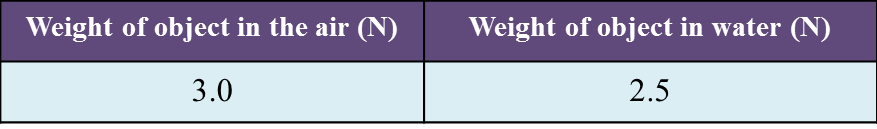(b)
The weight of object in the air is larger than the weight of object in the water.

(c)
The apparent loss in weight is equal to the weight of water displaced.

(d)
Weight of water displaced.

(e)
Upthrust is the apparent loss in weight of an object when it is immersed in water.

Question 2:
Diagram 2.1 shows the weight of a metal block in the air.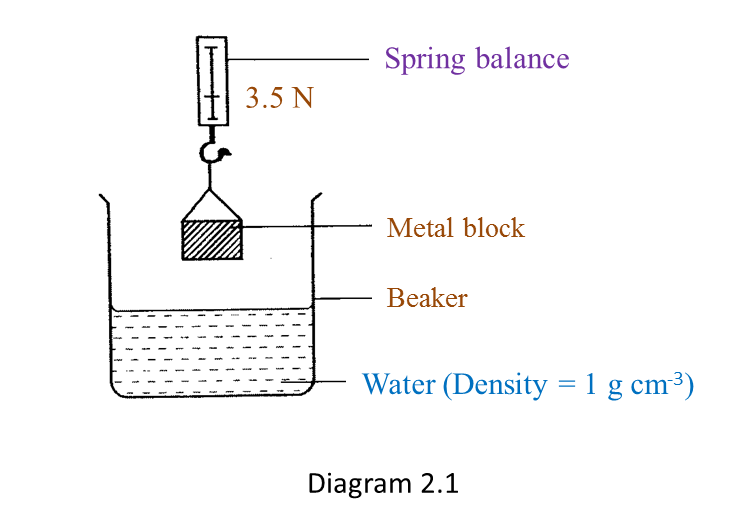Diagram 2.2 shows the apparent weight of the metal block when it is immersed in water.(a) Based on Diagram 2.1 and Diagram 2.2:
(i) State one observation from the result of this experiment. [1 mark]

(ii) State one inference from this experiment. [1 mark]

(b) State the constant variable in this experiment. [1 mark]

The experiment is repeated by using sea water as shown in Diagram 2.3.(c) Based on Diagram 2.3, what is the reading on the spring balance? [1 mark]

(d) Predict the reading of the spring balance if the metal block is immersed in a liquid which has higher density than sea water. [1 mark]

(a)(i)
The weight of the metal block in air is larger than the apparent weight of the same metal block immersed in water.

(a)(ii)
An upthrust acts on the metal block when it is immersed in water.

(b)
Metal block, water (density = 1 g cm-3). (any one)

(c)
2.4 N

(d)
The reading of the spring balance will be lower than the reading shown in Diagram 2.3.

# 5.7.2 The Concept of Pressure (Structured Questions)

Question 1:
Figure 1.1 shows an experiment to study the pressure produced by metal blocks, A and B of the same mass.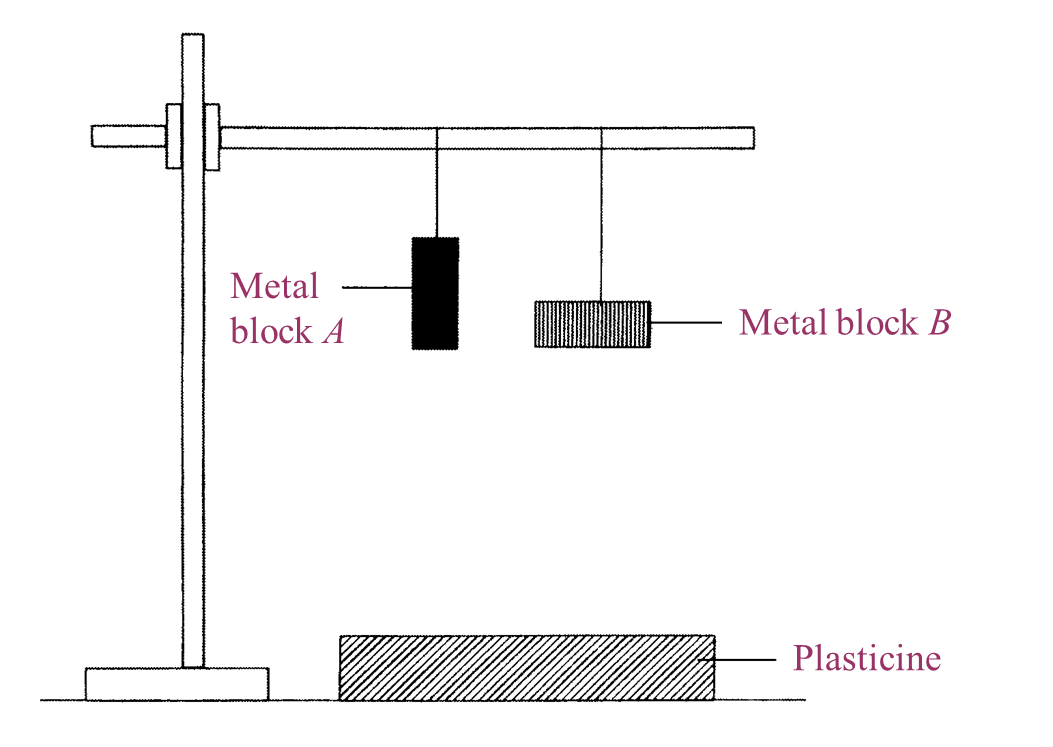Figure 1.1

Figure 1.2 shows the effect on the plasticine when metal blocks, A and B were dropped.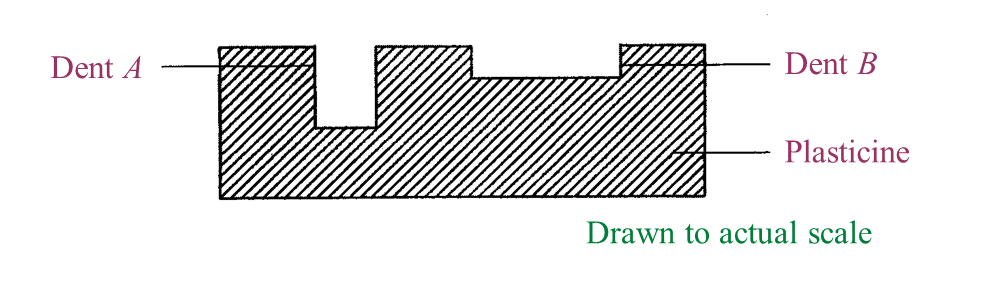Figure 1.2

(a)
What is the controlled variable in this experiment? [1 mark]

(b)
Write down one observation from the result of this experiment. [1 mark]

(c)
State one inference that can be made based on the observation in Figure 1.2. [1 mark]

(d)
Based on Figure 1.2, measure and record the depth of dent A. [1 mark]

(e)
Metal block C in Figure 1.3 has the same mass as metal blocks, A and B.Figure 1.3

Predict the depth of dent produced on the plasticine when metal block C is dropped from the same height. [1 mark]

(a)
The weight of the metal block (dropped from the same height).

(b)
The depth of dent A is more than the depth of dent B.

(c)
The surface area of A is smaller than the surface area of B.

(d)
The depth of dent A is 1 cm.

(e)
Deeper than dent A (or more than 1 cm).